# AVML 2012: ggplot2

–Josef Fruehwald, AVML, University of York, 2012

## Plotting principles

We are here to learn the basics of ggplot2. ggplot2 will be useful for producing complex graphics relatively simply. It won't be of any use for figuring out what is a sensible, useful, or accurate plot. To get a good handle on those, I'd advise simply reading a lot about data visualization.

## Installation

If you haven't already, take this opportunity to install the latest version of ggplot2.

install.packages("ggplot2")

library(ggplot2)


Right now, I'm running version 0.9.1, and some of the default behaviors of ggplot2 changed with version 0.9.0.

## Grammar of Graphics

ggplot2 is meant to be an implementation of the Grammar of Graphics, hence gg plot. The basic notion is that there is a grammar to the composition of graphical components in statistical graphics. By direcly controlling that grammar, you can generate a large set of carefully constructed graphics from a relatively small set of operations. As Wickham (2010), the author of ggplot2 said,

A good grammar will allow us to gain insight into the composition of complicated graphics, and reveal unexpected connections between seemingly different graphics

A good example of an unexpected connection would be that pie charts are just filled bar charts…

pie <- ggplot(mtcars, aes(x = factor(1), fill = factor(cyl))) +
geom_bar(width = 1, position = "fill", color = "black")
pie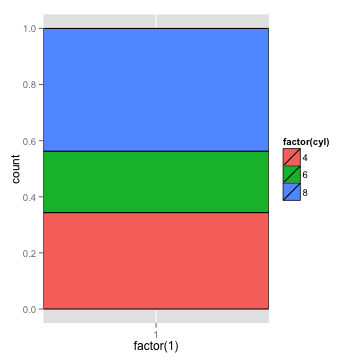…in polar coordinates.

pie + coord_polar(theta = "y")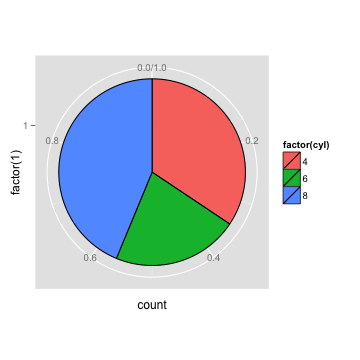## ggplot2 Materials

There are quite a few ggplot2 materials out there to guide you when you're not sure what to do next. First and foremost are the internal help pages for every piece of ggplot2 we'll cover here. They tend to be pretty useful.

?geom_jitter


## ggplot2 Basic Concepts

There are a few basic concepts to wrap your mind around for using ggplot2. First, we construct plots out of layers. Every component of the graph, from the underlying data it's plotting, to the coordinate system it's plotted on, to the statistical summaries overlaid on top, to the axis labels, are layers in the plot. The consequence of this is that your use of ggplot2 will probably involve iterative addition of layer upon layer until you're pleased with the results.

Next, the graphical properties which encode the data you're presenting are the aesthetics of the plot. These include things like

• x position
• y position
• size of elements
• shape of elements
• color of elements

The actual graphical elements utilized in a plot are the geometries, like

• points
• lines
• line segments
• bars
• text

Some of these geometries have their own specific aesthetic settings. For example,

• points
• point shape
• text
• text labels
• lines
• line weight
• line type

You'll also frequently want to plot statistics overlaid on top of, or instead of the raw data. Some of these include

• Smoothing and regression lines
• One and two dimensional binning
• Mean and medians with confidence intervals.

The aesthetics, geometries and statistics constitute the most important layers of a plot, but for fine tuning a plot for publication, there are a number of other things you'll want to adjust. The most common one of these are the scales, which encompass things like

• A logarithmic x or y axis
• Customized color scales
• Customized point shapes, or linetypes

The following sections are devoted to some of these basic elements in ggplot2.

### Layers

We'll be constructing plots with ggplot2 by building up “layers”. The layering of plot elements on top of each other is perhaps the most powerful aspect of the ggplot2 system. It means that relatively complex plots are built up of modular parts, which you can iteratively add or remove. For example, take this figure, which plots the relationship between vowel duration and F1 for 394 tokens of the lexical item “I”.

I_jean <- read.delim("http://bit.ly/avml_ggplot2_data")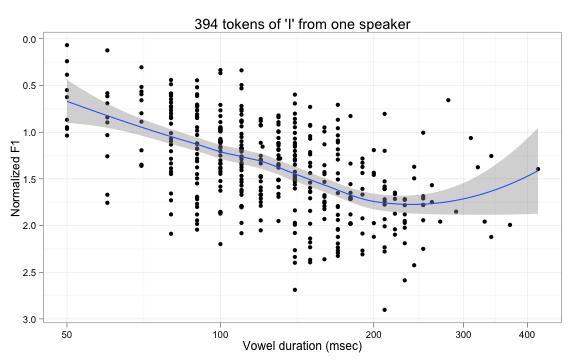This plot is composed of nine layers, which can be subdivided into five layer types.

#### The data layer

Every ggplot2 plot has a data layer, which defines the data set to plot, and the basic mappings of data to aesthetic elements. The data layer created with the functions ggplot() and aes(), and looks like this

ggplot(data, aes(...))


The first argument to ggplot() is a data frame (it must be a data frame), and its second argument is aes(). You're never going to use aes() in any other context except for inside of other ggplot2 functions, so it might be best not to think of aes() as its own function, but rather as a special way of defining data-to-aesthetic mappings.

For the plot from above, we'll be using data from the I_jean data frame, which looks like this:

head(I_jean)

##    Name Age Sex Word FolSegTrans Dur_msec     F1   F2
## 1 Jean   61   f  I'M           M      130  861.7 1336
## 2 Jean   61   f    I           N      140 1010.4 1349
## 3 Jean   61   f I'LL           L      110  670.1 1293
## 4 Jean   61   f  I'M           M      180  869.8 1307
## 5 Jean   61   f    I           R       80  743.0 1419
## 6 Jean   61   f I'VE           V      120  918.2 1581
##     F1.n    F2.n
## 1 1.6609 -0.8855
## 2 2.6883 -0.8536
## 3 0.3370 -0.9873
## 4 1.7168 -0.9536
## 5 0.8407 -0.6897
## 6 2.0512 -0.3068


I've decided that an interesting relationship in this data is between the vowel duration (Dur_msec) and the normalized F1 of the vowel (F1.n). Specifically, I'd like to map Dur_msec to the x-axis, and F1.n to the y-axis. Here's the ggplot2 code.

p <- ggplot(I_jean, aes(x = Dur_msec, y = F1.n))


Right off the bat, we can see one way in which ggplot2 is similar to lattice, and different from base graphics. If you've only ever used plot() in R, then you might be surprised to see me assigning the output of ggplot2 to an object, because plot() just creates a plot, not an object. ggplot2 plots, on the other hand, are objects, which you can assign, save, and manipulate.

If you try to print p right now though, you'll get an error. Right now, p is a ggplot2 plot that's all data, but no graphical elements. Adding graphical elements, or geoms is the next step.

#### The geometries layer

The next step, after defining the basic data-to-aesthetic mappings, is to add geometries to the data. We'll discuss geometries in more detail below, but for now, we'll add one of the simplest: points.

p <- p + geom_point()
p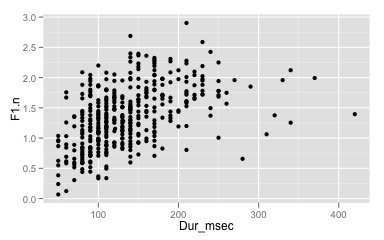There are a few things to take away from this step. First and foremost, the way you add new layers, of any kind, to a plot is with the + operator. And, as we'll see in a moment, there's no need to only add them one at a time. You can string together any number of layers to add to a plot, separated by +.

The next thing to notice is that all layers you add to a plot are, technically, functions. We didn't pass any arguments to geom_point(), so the resulting plot represents the default behavior: solid black circular points.

If for no good reason at all we wanted to use a different point shape in the plot, we could specify it inside of geom_point().

ggplot(I_jean, aes(x=Dur_msec, y=F1.n)) +
geom_point(shape = 3)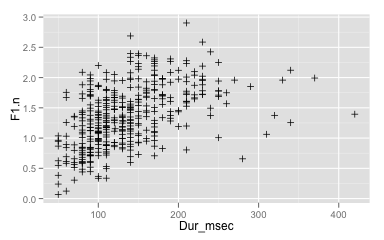Or, if we wanted to use larger, red points, we could specify that in geom_point() as well.

ggplot(I_jean, aes(x=Dur_msec, y=F1.n)) +
geom_point(color = "red", size = 3)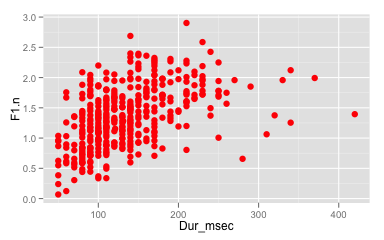Speaking of defaults, we can see a few of the default setting of ggplot2 on display here. Most striking is the light grey background, with white grid lines. Opinion varies on whether or not this is aesthetically or technically pleasing, but don't worry, it's adjustable.

Another default is to label the x and y axes with the column names from the data frame. I'll inject a bit of best practice advice here, and tell you to always change the axis names. It's nearly guaranteed that your data frame column names will make for very poor axis labels. We'll cover how to do that shortly.

Finally, note that we didn't need to tell geom_point() about the x and y axes. This may seem trivial, but it's a really important, and powerful aspect of ggplot2. When you add any layer at all to a plot, it will inherit the data-to-aesthetic mappings which were defined in the data layer. We'll discuss inheritance, and how to override, or define new data-to-aesthetic mappings within any geom.

#### The statistics layer

The final figure also includes a smoothing line, which is one of many possible statistical layers we can add to a plot.

p <- p + stat_smooth()
p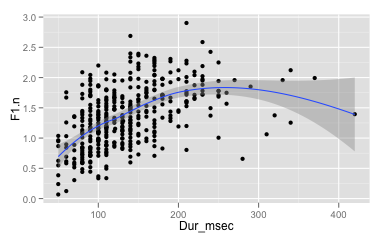We'll go over the default behavior of stat_smooth() below, but in this plot, the smoothing line represents a loess smooth, and the semi-transparent ribbon surrounding the solid line is the 95% confidence interval.

One important thing to realize is that it's not necessary to include the points in order to add a smoothing line. Here's what the plot would look like with the points omitted.

ggplot(I_jean, aes(x = Dur_msec, y = F1.n))+
stat_smooth()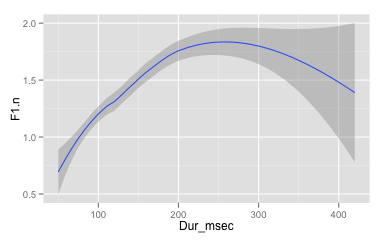Notice how the y-axis has zoomed in to just include the range of the smoothing line and standard error.

#### Scale transformations

I also wanted to make some alterations to the default x and y axis scales. For example, the y-axis is currently running in reverse to the intuitive direction of F1. Higher vowels have lower F1 values, so we want to flip the y-axis. Additionally, durations are typically best displayed along a logarithmic scale, so we should convert the x-axis as well.

p <- p + scale_x_log10(breaks = c(50, 100,200,300,400))+
scale_y_reverse()
p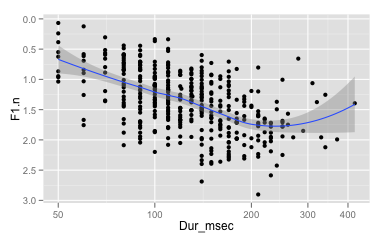It's worth noting that the smoothing line here is calculated over the transformed data.

#### Cosmetic alterations

Finally, I wanted to make some cosmetic adjustments to the plot. For example, the x-axis label “Dur_msec” is not quite as useful as “Vowel duration (msec)” would be. I also added a title to the plot, and changed the color theme to black and white.

p <- p + ylab("Normalized F1")+
xlab("Vowel duration (msec)")+
theme_bw()+
opts(title = "394 tokens of 'I' from one speaker")
p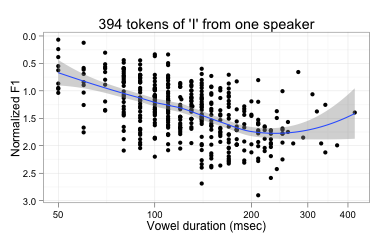Here's all the layers, put together all at the same time.

ggplot(I_jean, aes(x=Dur_msec, y=F1.n))+
geom_point()+
stat_smooth()+
scale_x_log10(breaks = c(50, 100,200,300,400))+
scale_y_reverse()+
ylab("Normalized F1")+
xlab("Vowel duration (msec)")+
theme_bw()+
opts(title = "394 tokens of 'I' from one speaker")### Aesthetics

In ggplot2, aesthetics are the graphical elements which are mapped to data, and they are defined with aes(). To some extent, the aesthetics you need to define are dependent on the geometries you want to use, because line segments have different geometric properties than points, for example. However, there is also a great deal of uniformity in the aesthetics used across geometries. Here is a list of the most common aesthetics you'll want to define.

• x
• x-axis location
• y
• y-axis location
• color
• The color of lines, points, and the outside borders of two dimensional geometries (polygons, bars, etc.). Hadley Wickham, the primary ggplot2 developer, is from New Zealand, so colour is also supported!
• fill
• The fill color of two dimensional geometries.
• size
• The size of points, or the weight of lines and borders of two dimensional geometries.
• shape
• This is specific to points, and defines the point shape. This is one of the few aesthetics to which you can't map a continuous variable.
• linetype
• This defines the line type of any kind of line, path, or border of a two dimensional geometry. This is another aesthetic which cannot be mapped to a continuous variable.
• alpha
• This defines the opacity of any geometric property. It's less commonly mapped to data, and more often hard coded to a single value as a solution for overplotting.
• xend, yend
• You'll use these more rarely, usually when plotting a line segment, or arrow. The beginning of the line segment will be located at x, y, and the end of the line segment will be located at xend,yend.
• ymin, ymax, (xmin, xmax)
• ymin and ymax are reserved for geometries which are devoted to representing ranges of data, like error bars, and ribbons. For the most part, these will be expressed along the y-axis, but xmin and xmax are utilized for some geometries as well.

The most important thing to keep in mind about aesthetics is not what they're called, though, but how they are inherited by the layers. Let's start by mapping the Word to color. 88% of the tokens are just “I”, so lets create a subset of the data that excludes “I” so it doesn't visually swamp the plot.

I_subset <- subset(I_jean, Word != "I")

ggplot(I_subset, aes(Dur_msec, F1.n, color = Word))+
geom_point()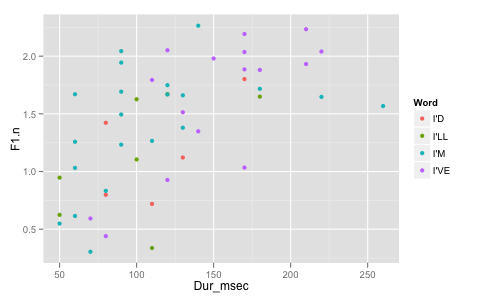Each point is now colored according to the word it corresponds to. ggplot2 has automatically generated a color palette of the right type and size, based on the data mapped to color, and created a legend to the side. As with everything, the specific color palette we use is adjustable, which will be discussed in more detail below under Scales. The default ggplot2 color palette is rather clever, however. Every color is equidistant around an HSL color circle, and have equal luminance. The idea is that no category should be accidentally visually emphasized, but they can be hard for some colorblind readers, and they will all print to the same shade of grey!

But on to more pressing matters. The only possible way to map the Word data to the color of points in the plot is to do it within aes(). If you are used to working with base graphics, then a lot of your instincts are wrong for ggplot2. For example, you might think the following would work:

I_subset$Color <- c("black", "red","blue", "green","goldenrod")[I_subset$Word]

ggplot(I_subset, aes(Dur_msec, F1))+
geom_point(color = I_subset$Color)  ## Error: When _setting_ aesthetics, they may only take one ## value. Problems: colour  The error is yelling at us about the distinction between setting an aesthetic, and mapping an aesthetic. Recall from above that we were able to set the color of all points to red by saying so inside of aes(), ggplot(I_subset, aes(Dur_msec, F1))+ geom_point(color = "red")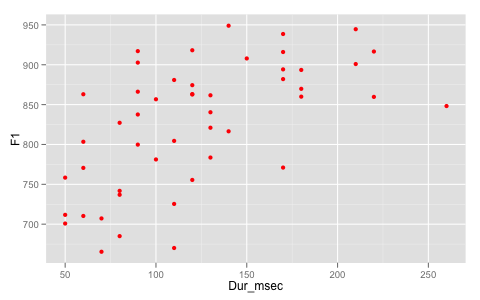But that's as far as you can go with that. Everything beyond setting a single color for all points in a plot constitutes mapping colors, and you have to use aes() for that. But mapping data to specific color values is still not as simple as you might initially think. For example, mapping Color to color inside of aes() produces this stroop test. ggplot(I_subset, aes(Dur_msec, F1, color = Color))+ geom_point()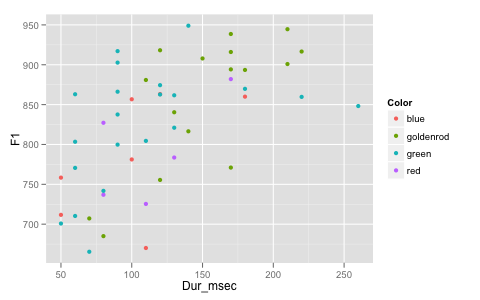The lesson here is not to try this way of constructing your own custom color palettes. We'll go over how to construct custom palettes under Scales. #### Inheritance If we add one more geometry (a line), we see that it also inherits the mapping of Word to color. ggplot(I_subset, aes(Dur_msec, F1.n, color = Word))+ geom_point()+ geom_line()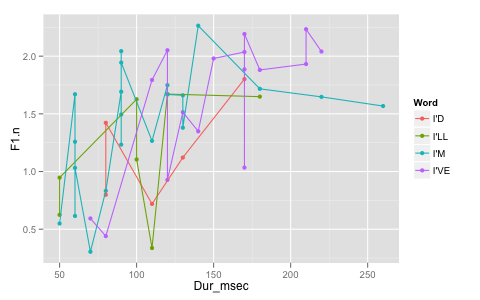There are a few important things to take note of in this plot. First, you can see that we have actually added four lines to the plot, one for each color. In most cases, when you map categorical data to an aesthetic like color, you are also defining sub-groupings of the data, and ggplot2 will draw a lines, calculate statistics, etc. separately for every sub-grouping of the data. The second important thing to notice is that geom_line() joins up points as they are ordered along the x-axis, not according to their order in the original data frame. There is a geom which will join up points that way called geom_path(). They point here, though, is that it is possible to define data-to-aesthetic mappings inside of geom functions, also by using aes(). Here, instead of mapping Word to color inside of ggplot(), we'll do it inside of geom_point(). ggplot(I_subset, aes(Dur_msec, F1.n))+ geom_point(aes(color = Word))+ geom_line()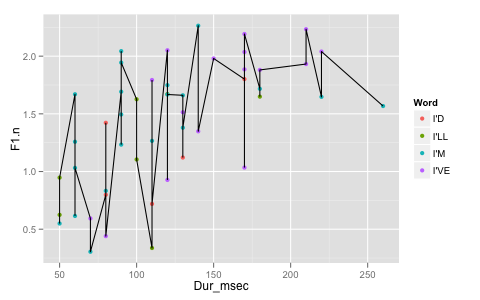The points are still colored according to the word, but there is only one, black line. We can also try passing aes(color = Word) to geom_line(). ggplot(I_subset, aes(Dur_msec, F1.n))+ geom_point()+ geom_line( aes(color = Word))+ scale_color_hue(direction = -1)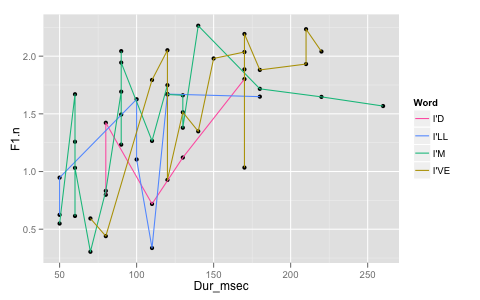Now, the lines are colored according to the word, but the points are all black. This brings up the all important point about aesthetics: Geoms inherit aesthetic mappings from the ggplot() data layer, and not from any other layer. #### Grouping Let's look at the effect of mapping Word to color on the calculation of statistics, like smoothing lines. Note, inside of stat_smooth() I've said se = F to turn off the display of standard errors. ggplot(I_subset, aes(Dur_msec, F1.n, color=Word))+ geom_point()+ stat_smooth(se = F)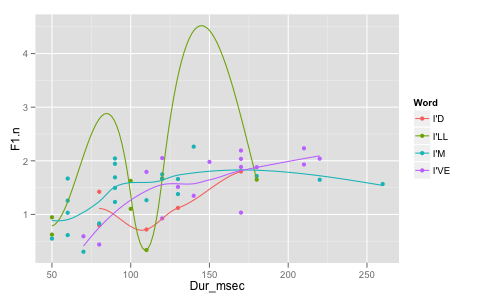Just like separate lines were drawn for each group as defined by color=Word, ggplot2 has calculated separate smoothers for each subset. If we had only passed color=Word to geom_point(), though, stat_smooth() would not have inherited that mapping, resulting in a single smoother being calculated.  ggplot(I_subset, aes(Dur_msec, F1.n))+ geom_point(aes(color=Word))+ stat_smooth(se = F)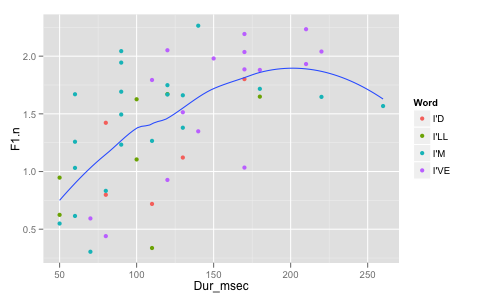It's important to understand that when you map categorical variables to an aesthetic that you're also defining sub-groupings. For example, if we map Word to shape, instead of color, the point shapes will now represent the word. ggplot(I_subset, aes(Dur_msec, F1.n, shape=Word))+ geom_point()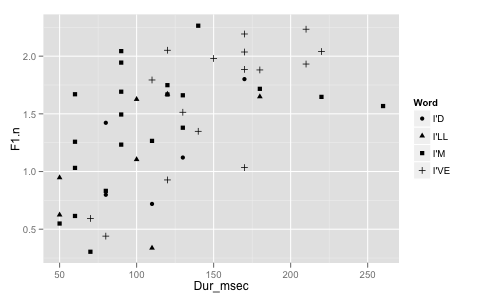Now if we add a smoother to this plot, even though shape isn't defined for lines, the smoother will still plot a different smoothing curve for each sub-grouping. ggplot(I_subset, aes(Dur_msec, F1.n, shape=Word))+ geom_point()+ stat_smooth(se = F)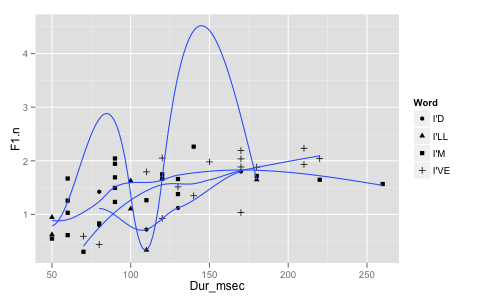If you really only wanted a single smoother line for all of the data in this case, one solution would be to move the shape=Word mapping from the data layer to the geom_point() layer. But in most cases, it's actually more desirable to override the aesthetic mapping. We can do this with the special aesthetic group. group does exactly what it sounds like it ought to: it defines groups of data. When you want to override groups defined in the data layer, you can do so by saying group=1. ggplot(I_subset, aes(Dur_msec, F1.n, shape=Word))+ geom_point()+ stat_smooth(se = F, aes(group = 1))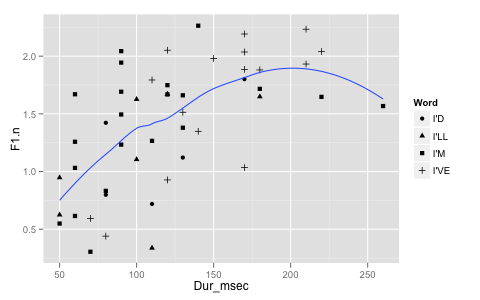The effect it has on stat_smooth() is that just a single smoother is calculated. If we come back to color = Word, and then draw a line with group = 1, the effect is that we draw one line that varies in color. ggplot(I_subset, aes(Dur_msec, F1.n, color=Word))+ geom_line(aes(group = 1))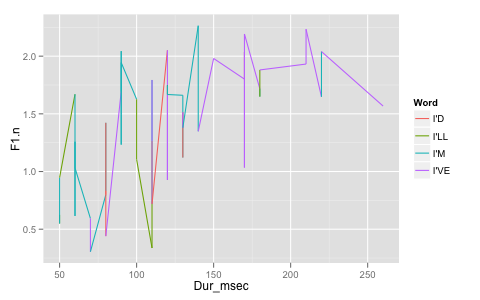#### More aesthetics and their use. So far, we've only mapped categorical variables to color, but it's also possible to map continuous variables to color. Here we'll redundantly map F1.n to both y and color. ggplot(I_jean, aes(Dur_msec, F1.n, color = F1.n))+ geom_point()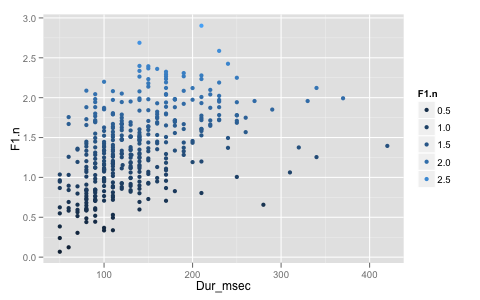Another important aesthetics distinction is between color and fill. If we wanted to create a bar chart of word frequencies, we could do so by mapping Word to the x-axis, and adding geom_bar() without any y-axis variable defined. ggplot(I_jean, aes(Word))+ geom_bar()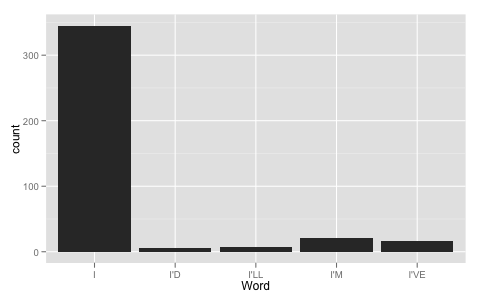If you also wanted to color the bars according to the word, your first instinct would probably be to map color = Word. But the result is that only the colors of the bars' outlines are mapped to Word. ggplot(I_jean, aes(Word, color = Word))+ geom_bar()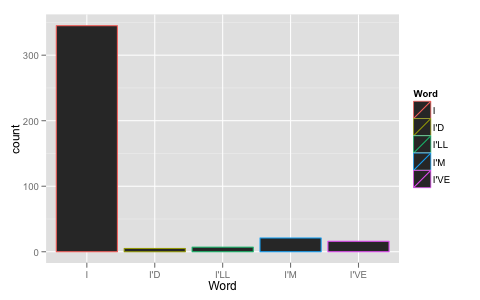What is probably more advisable is to map Word to fill, which control the filling color of two dimensional geoms. ggplot(I_jean, aes(Word, fill = Word))+ geom_bar()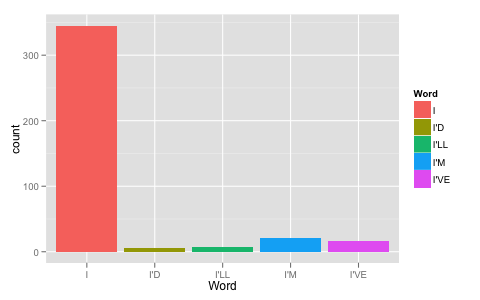As you might have figured out now, it's technically possible to map the fill color of bars to one variable, and the outline color to different variable. My advice is to never do such a thing, because the results almost always come out a jumbled mess. Instead, I would suggest setting the color of the bars to black. I find it more pleasing to the eye, and helps to emphasize the divisions between bars when they're stacked. Compare this plot: ggplot(I_subset, aes(Name, fill = Word))+ geom_bar()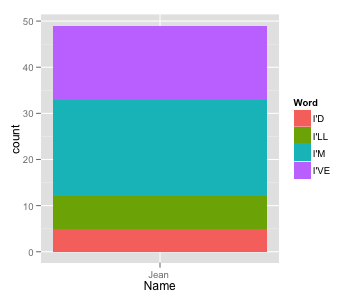to this one. ggplot(I_subset, aes(Name, fill = Word))+ geom_bar(color = "black")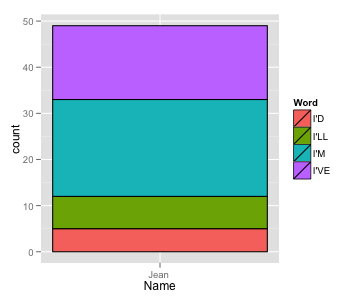### Geometries So far, we've used the following geometries: • geom_point() • geom_line() • geom_bar() All geometries begin with geom_, meaning you can get a full list using apropos(). apropos("^geom_")  ##  "geom_abline" "geom_area" "geom_bar" ##  "geom_bin2d" "geom_blank" "geom_boxplot" ##  "geom_contour" "geom_crossbar" "geom_density" ##  "geom_density2d" "geom_dotplot" "geom_errorbar" ##  "geom_errorbarh" "geom_freqpoly" "geom_hex" ##  "geom_histogram" "geom_hline" "geom_jitter" ##  "geom_line" "geom_linerange" "geom_map" ##  "geom_path" "geom_point" "geom_pointrange" ##  "geom_polygon" "geom_quantile" "geom_raster" ##  "geom_rect" "geom_ribbon" "geom_rug" ##  "geom_segment" "geom_smooth" "geom_step" ##  "geom_text" "geom_tile" "geom_violin" ##  "geom_vline"  This is a quite extensive list, and we won't be able to cover them all today. Many of them are actually convenience functions for special settings of other geoms. For example, geom_histogram() is really just geom_bar() with special settings. ggplot(I_jean, aes(F1.n))+ geom_histogram()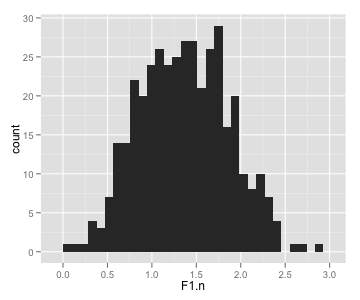Other geoms are just convenience functions for statistical layers. For example, you'll notice geom_smooth(), which if you add it to a plot will have the same behavior of stat_smooth(), which we've already been using extensively. ggplot(I_jean, aes(Dur_msec, F1.n))+ geom_smooth()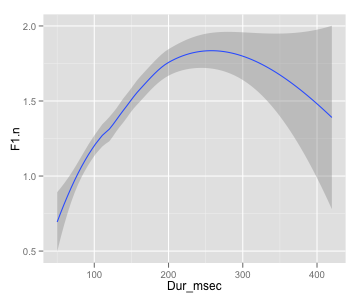#### Some special geoms Some geoms are both unique and common enough in their usage to warrant special mention. ##### geom_line() vs geom_path() As I said above, when you add geom_line() to a plot, it connects points up according to their order along the x-axis. If you want to connect points according to their order in a data frame (say, to illustrate a trajectory through two-dimensional space over time), you should use geom_path(). mod_F1 <- loess(F1.n ~ Dur_msec, data = I_jean) mod_F2 <- loess(F2.n ~ Dur_msec, data = I_jean) pred <- data.frame(Dur_msec = seq(50, 400, length = 100)) pred$F1.n <- predict(mod_F1, newdata = pred)
pred\$F2.n <- predict(mod_F2, newdata = pred)

ggplot(pred, aes(-F2.n, -F1.n, color = Dur_msec))+
geom_path()+
geom_point()